# Harmonic Filter Design to Mitigate Two Resonant Points in a Distribution Network

Author: Sybrand Bester (E-mail: bestersj@gmail.com) and Gary Atkinson-Hope (Email: atkinsonhopeg@cput.ac.za) Cape Peninsula University of Technology, Belville 7539, South Africa

Corresponding author: Gary Atkinson-Hope, Ph.D., research fields: VDC and harmonic analysis, computational studies. E-mail: atkinsonhopeg@cput.ac.za.

Journal of Energy and Power Engineering 6 (2012) 2018-2023

1. Introduction

The increasing unease over harmonic distortions stems from the installation and application of highly non-linear power electronic devices, like VSD (variable speed drives) to control power apparatus in the industry .

When supplied by a voltage of 50 Hz, a non-linear load draws harmonic currents that penetrate networks causing waveform distortions . Inductive loads absorb reactive power. Installing parallel capacitors will improve the power factor, because they provide reactive power . Bus voltages decrease due to increasing of load demand. It is common to strategically place capacitor banks to help raise the voltage profile back to nominal values . The capacitors used to increase the bus voltage and improve the power factor can have harmful effects on the network when harmonics are present in the system as they can cause harmonic resonance to occur.

Parallel resonance occurs when the magnitudes of the capacitive and inductive reactance are equal. If the parallel resonant peak is aligned with the frequency of a characteristic harmonic injected by the non-linear load, high voltage and currents can flow which can cause damage to equipment in the network. The IEEE Std. 519-1992 was created to help the user keep these voltage and current distortions within an acceptable level at the PCC (point of common coupling).

The escalating levels of distortion in distribution systems can be best contained by the installation of harmonic filters at strategic locations . The most commonly used harmonic filters are the high-pass filter and the single tuned filter. Refs. [6-8] discuss how voltage and current distortions are brought down to acceptable levels according to the IEEE Std. 519-1992 using passive filters.

While all these cases investigate a situation where there is one parallel resonant point caused by a capacitor, none of them relates a case where there are two shunt capacitors situated in the network causing two parallel resonant points to occur. For this reason, it was deemed necessary to create a network using simulation software that can conduct harmonic studies and could evaluate when parallel resonance occurs if capacitors are installed for power factor correction and bus voltage regulation and then come up with the best solution to reduce the distortions caused by the resonance using harmonic filters. The solutions must ensure that the system is within the IEEE Std.519-1992 voltage and current limitations.

2. Research Statement

The purpose of this research is to investigate a scenario where two resonant points occur in a system caused by power capacitors. The bus voltage is purposefully lowered below the NRS 048-2:2004 voltage limit of 6% so that the shunt capacitor added to improve the voltage limit to the nominal value together with the power factor capacitor connected at the consumer bus causes resonance in the system . An additional objective is to design single-tuned and high-pass harmonic filters to reduce the harmonic distortions caused by the parallel resonance because of multiple resonant points. The voltage and current distortions must not exceed IEEE Std. 519-1992 limitations at the PPC.

3. Methodology

The network was simulated in DIgSILENT 14 power factory software. Harmonic studies were first conducted without capacitors and then with capacitors present in the network. High pass filters and single-tuned filters were installed at two different points in the network. During each of the different scenarios, the system was checked to determine at which characteristic harmonic order resonance occurred. The voltage and current distortions at the PCC were also observed to see whether it fell within the IEEE Std. 519-1992.

4. Theoretical Background

4.1 Busbar Voltage Regulation

The bus voltages were decreased below NRS 048-2:2004 voltage limit as shown in Table 1 .Table 1 NRS 048-2 voltage limits.

4.2 Harmonic Filter Design

(1) Capacitor size

The size of the capacitor for the filters should be determined by the reactive power needed to increase the busbar power factor. The following equation is used to describe the relationship between active and reactive power when deciding the value of capacitance: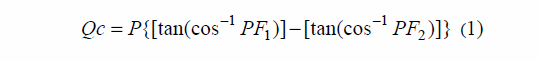Qc is the total capacitive reactive power. PF1 is the power factor before the capacitor is added and PF2 is the power factor after the capacitor is added. P is the real power.Real power is calculated by:*

where: V = phase voltage, I = phase current; P = real power and Q = reactive power.

(2) Single-tuned filter

This type of filter consists of a capacitor in series with a reactor. The single-tuned filter is good for trapping a specific harmonic. It gives a portion or all the reactive power required for power factor correction .

(3) High-pass filter

The filter consists of a capacitor in series with a combination of a resistor and a reactor. A high pass filter is one through which the higher frequencies pass .

(4) Filter design equations The formulas listed below can be used to design a high-pass filter and a single-tuned filter. Capacitive reactance (XC):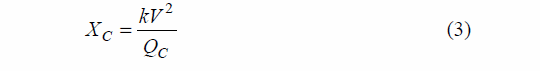Inductive reactance (XL): X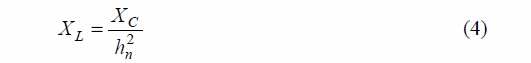Characteristic reactance (Xn):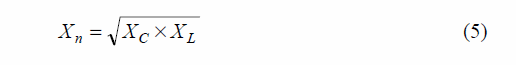Reactive power of the filter (QF):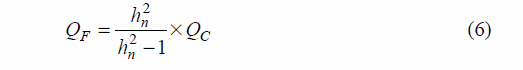Single-tuned resistor (RS):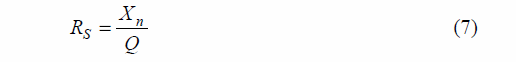High-pass resistor (RH):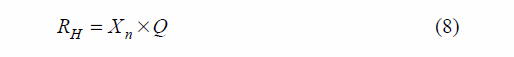where: hn = Tuning order; Q = Quality factor.

(5) Quality-factor

The Q-factor (quality factor) is determined by the value of the filter resistor and determines the sharpness of the frequency to which it is tuned . The Q-factor of a single-tuned filter is normally between 30 and 100 . Harmonic filters with a low Q-factor like the high-pass filter have a Q-factor between 0.5 and 5 .

(6) Selection of filter tuning order

Filters are tuned 3%-15% below the harmonic order being filtered .

4.3 Calculations for Harmonic Distortion Limits

Individual harmonic voltage distortion (HDV):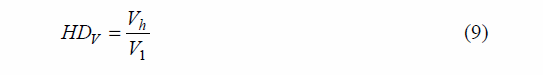Total harmonic voltage distortion (THDV):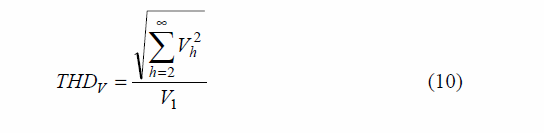where: Vh = harmonic voltage, V1 = voltage at fundamental frequency (f1 = 50 HZ).

Individual harmonic current distortion (HDI)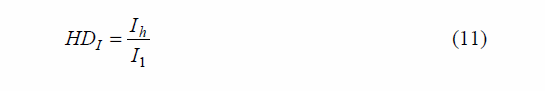Total demand distortion (TDDI):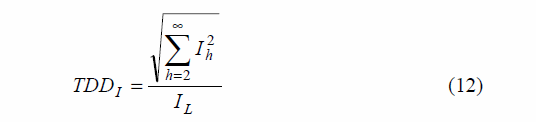where:

Ih = harmonic current magnitude;

IL = fundamental component maximum demand load current;

I1 = current at fundamental frequency (f1 = 50 HZ).

SCR (short circuit ratio):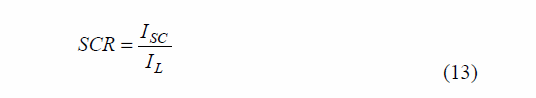where: ISC = short circuit current at the PCC.

4.4 IEEE Std. 519-1992 Limitations

The standard recommends restrictions for harmonic distortions measured at the point of common coupling . The most amount of harmonic current distortion a consumer can inject into the utility network is specified by the limit in Table 2 [14, 15]. Only the data needed to examine distortion limits at the PCC are shown in Tables 2 and 3.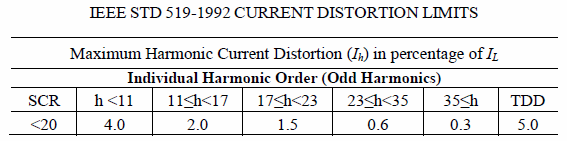Table 2 IEEE STD 519-1992 current distortion limits.

The supply of a clean undistorted voltage to the consumers is the responsibility of the utility . The customers can only be guilty of distorting the voltage if they do not meet the harmonic current limits . The harmonic voltage limits are shown in Table 3 .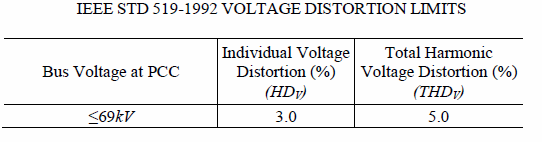Table 3 IEEE STD 519-1992 voltage distortion limits.

5. One-Line Diagram

The network investigated was modelled in DIgSILENT 14 power factory software. The network consists of three loads; one is non-linear (VSD) with a harmonic spectrum shown in Table 4.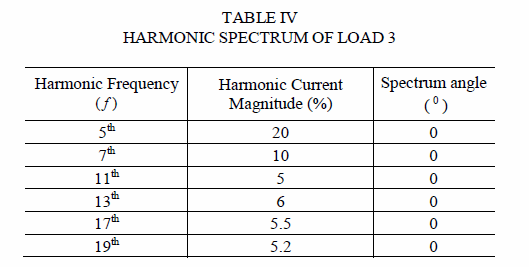Table 4 Harmonic spectrum of load 3.

BUS 3 is the PCC and the network parameters are given in Fig. 1.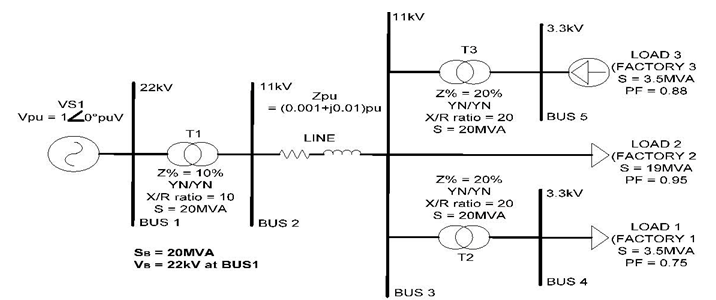6. Simulation Cases

Case study 1

The network in Fig. 1 was modelled, simulated and the PCC was observed. The power factor of the customers and the voltage at each bus was also recorded.

Case study 2

A capacitor with the value of 8.6 MVAr was connected at BUS 2 to increase the bus voltages of BUS 2 and BUS 5 and increase the power factor of the system from 0.92 to 0.99. Another capacitor (0.9 MVAr) was connected in parallel with load 3 to increase the power factor from 0.88 to 0.97.

Case study 3

A single-tuned filter was designed using the capacitor at BUS 2. The filter was tuned 5% below the 5th harmonic order i.e. 4.75th. The main idea was to avoid harmonics rather than decrease a specific one. The capacitor at BUS 5 was still connected.

Case study 4

The single-tuned filter was removed in case study 3 and replaced with a 4.75th harmonic high-pass filter.  This filter was also designed to avoid harmonics.

Case study 5

The filter at BUS 2 in case study 4 was replaced with the 8.6 MVAr capacitor and a 4.75th harmonic single-tuned filter was designed from the power factor correction capacitor at BUS 5.

Case study 6

The single-tuned filter from case study 5 was replaced with a 4.75th harmonic high-pass filter.

The short circuit ratio was found to be in the < 20 category for all cases. The results were recorded at the PCC.

7. Results

Capacitors caused two resonances near 5th and 11th.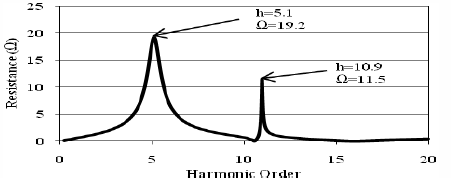Figure 2. Impedance scan of Case Study 2 performed at the PCC

Other harmonics i.e. 7th, 13th, 17th and 19th fell within the IEEE Std. 519 limits. In Fig. 2, the percentage distortions (HDV) caused by the 5th and 11th harmonic currents can be seen. Fig. 3 shows the total demand distortions (TDDI) at PCC.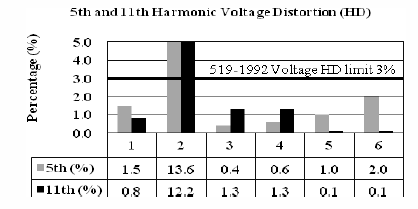Figure 3. Comparison of 5th and 11th (HDV) results of case studies 1-6

Total voltage distortion (THDV) calculated from voltages at the PCC is shown in Fig. 4.

Figure 4 shows the total demand distortions (TDDI) at PCC.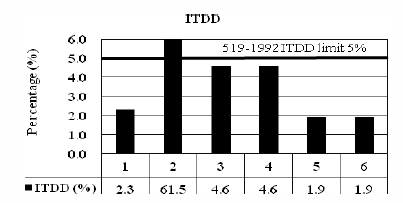Figure 4. Comparison of TDDI results of case studies 1-6

Total voltage distortion (THDV) calculated from voltages at the PCC is shown in figure 5: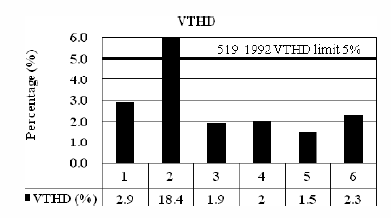Figure 5. Comparison of THDV results of case studies 1-6

Impedance scans were taken from case studies 2, 5 and 6.  The difference in impedance can be seen in Fig. 6.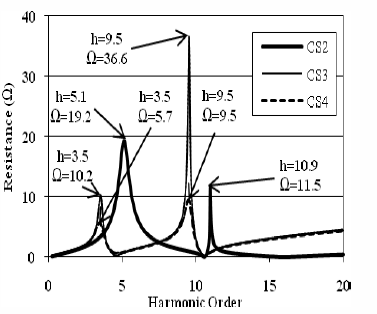Figure 6. Comparison of Impedance scans of Case Study 2, 3 and 4, performed at the PCC

Impedance scans were taken of Case Study 2, 5 and 6. The difference in impedance can be seen in figure 7: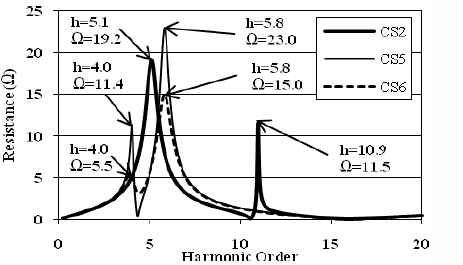Figure 7. Comparison of Impedance scans of Case Study 2, 5 and 6, performed at the PCC

8. Analysis of Results

Case studies 1 and 2

It can be seen from case study 1 that the network was next to no harmonic distortion in the network, before the power factor capacitors and the capacitor to increase the bus voltage were added. The capacitors added in case study 2 caused parallel resonance to occur near the 5th and 11th harmonic order, seen in Fig. 7. The 5th and 11th currents injected by load 3 caused an interaction between the resonant points and the currents and caused a harmonic resonance to occur. This increased the 5th and 11th voltage distortions and the 11th current distortions, including the total demand distortion above the IEEE Std. 519-1992 limitations.

Case study 3 and 4

The single-tuned and high-pass filters performed almost exactly the same due to the distortion levels they decreased as shown in Figs. 1 and 2. The resonant points were also successfully moved seen in Fig. 5, and there was success at increasing the bus voltages of the system and improving the power factor.

Case studies 5 and 6

Harmonic filters performed the same as the filters in case studies 3 and 4, also due to the decreased distortions shown in Figs. 2-4 and 7 that were almost exactly the same. The resonant peaks were also successfully moved as shown in Fig. 6. The filters further provided the power factor needed for the customer connected to BUS 5.

9. Conclusions

This paper has presented the application of single-tuned and high-pass harmonic filters at the utility and at the consumer end when two parallel resonant points are present in the system. Installing harmonic filters at the customer side to remove harmonic distortions proved to be more effective in meeting the harmonic distortion limits when looking at the PCC. This paper also proved that harmonic penetration studies can be performed using DIgSILENT power software. It is recommended that more research should be done when there are three or multiple resonant points present in the network that resonate with characteristic harmonic numbers and to see how harmonic filters would decrease distortion levels when unacceptable distortions are observed at the PCC.

Acknowledgments

This work was supported by the Electricity Supply Commission of South Africa (Eskom) under a Tertiary Education Support Grant 2010.

References

     Y.T. Hsiao, Design of filters for reducing harmonic distortion and correcting power factor in industrial distribution systems, Tamkang Journal of Science and Engineering 4 (3) (2001) 193-199.

     R.S. Vedam, M.S. Sarma, Power Quality: VAR Compensation in Power Systems, CRC Press, Boca Raton, 2009, pp. 6-7.

 R. Natarajan, Power System Capacitors, Taylor and Francis Group, Singapore, 2005, pp. 35-45.

 F.C. de la Rosa, Harmonics and Power Systems, Taylor and Francis Group, Boca Raton, 2006, pp. 85-86.

 J. Arrillaga, N.R. Watson, Power System Harmonics, 2nd ed., Wiley, West Sussex, 2003, pp. 245-246.

 E.B. Makram, E.V. Subramaniam, A.A. Girgis, R. Catoe, Harmonic filter design using actual recorded data, IEEE Trans. Ind. Appl. 29 (1993) 1176-1183.

 M.E. de Lima Tostes, U.H. Bezerra, R.D.S. Silva, J.A.L. Valente, C.C.M. de Moura, T.M.M. Branco, Impacts over the adoption distribution grid from the adoption of distributed harmonic filters on low-voltage customers, IEEE Trans. Power Del. 20 (2005) 384-389.

 P.J. McQueen, C. Stemmet, Harmonic analysis and mitigation for an industrial plant, in: Btech Conference, Nov. 13, 2009, Vol.13, pp. 9-14.

 Electricity Supply—Quality of Supply—Part 2: Voltage Characteristics, Compatibility Levels, Limits and Assessment Methods, 2004, pp. 13-17.

 G.W. Wakileh, Power System Harmonics Fundamentals, Analysis and Filter Design, Springer, New York, 2001.

 A.B. Nassif, W. Xu, W. Freitas, An investigation on the selection of filter topologies for passive filter applications, IEEE Trans. Power Del. 24 (3) (2009) 1710-1718.

  IEEE Std.1531-2003, IEEE Guide for Application and Specification of Harmonic Filter, 2003.

 Variable frequency drive: Operation and application of variable frequency drive (VFD) technology [Online], Carrier Corporation, http://www.carriercca.com/Supporting_Doc/Water_Coole d_Chillers/wp_varfreqdrive.pdf.

 T.M. Blooming, D.J. Carnovale, Application of IEEE STD 519-1992 harmonic limits, in: Pulp and Paper Industry Technical Conference, WI, USA, June 18-23, 2006, pp. 1-9.

 IEEE Std.519-1992, IEEE Recommended Practice and Requirements for Harmonic Control in Electrical Power Systems, 1993.